# Sequence Alignment

#### What is Alignment?

Let's distinguish between two uses of "alignment":

• Informal:
• A comparison of two potentially related sequences.
• Usually by hand.
• Example:
```
C T C T A G C A T T A G
G T G C A C C C A
```
• Prone to error for large sequences.
• Not systematic or quantitative.

• Formal:
• Precise operators for alignment: matching, gaps
Example:
```
C T C T A G C A T T A G
G T - G C A C C C A     (insert a gap for better match)
```
• Quantitative scoring system for matches and gaps.
• Systematic search among possible alignments.
• Use alignment algorithms to find optimal alignment.
• Different alignment algorithms (global, local, affine-penalty).

Types of two-sequence alignments:

• Global alignment:
• Input: treat the two sequences as potentially equivalent.
• Goal: identify conserved regions and differences.
• Algorithm: Needleman-Wunsch dynamic programming
• Applications:
• Comparing two genes with same function (in human vs. mouse).
• Comparing two proteins with similar function.

• Local alignment:
• Input: The two sequences may or may not be related.
• Goal: see whether a substring in one sequence aligns well with a substring in the other.
• Algorithm: Smith-Waterman dynamic programming
• Applications:
• Searching for local similarities in large sequences (e.g., newly sequenced genomes).
• Looking for conserved domains or motifs in two proteins.

• Semi-global alignment:
• Input: two sequences, one short and one long.
• Goal: is the short one a part of the long one?
• Algorithm: modification of Smith-Waterman.
• Applications:
• Given a DNA fragment (with possible error), look for it in the genome.
• Look for a well-known domain in a newly-sequenced protein.

• Suffix-prefix alignment:
• Input: two sequences (usually DNA)
• Goal: is the prefix of one the suffix of the other?
• Algorithm: modification of Smith-Waterman.
• Applications:
• DNA fragment assembly.

• Heuristic alignment:
• Input: two sequences.
• Goal: See if two sequences are "similar" or candidates for alignment.
• Algorithms: BLAST, FASTA (and others)
• Applications:
• Search in large databases.

Difference between keyword-search and alignment:

• Keyword search:
• Keyword search is exact matching.
• Can be done quickly (straightforward scan).
• Used in Entrez (for example)

• Alignment:
• Non-exact, scored matching.
• Takes much more time.
• Used in tools like BLAST2, CLUSTALW.

Problems from a biologist's point of view:

• "I have just sequenced a DNA fragment"
=> you have a piece of unknown DNA.
• Run a BLAST search (which uses the BLAST algorithm).
=> result: other DNA fragments (or genomes) that contain similar stretches.
• Once you have candidates, run a more careful alignment among them.
=> local alignment or semi-global alignment.

• "I've located a gene using a gene-finding algorithm"
=> you have the gene sequence fairly well determined.
• Run BLAST to locate similar genes.
• Run a global alignment to see differences.
• Alternatively, identify potential proteins via a protein database.

• "I'm confirming a sequencing experiment first done by someone else"
=> do a global alignment.

• "I've sequenced a new protein"
=> BLAST search (on proteins) and then global alignment on results.

• "I've found a new domain or motif"
=> BLAST to locate proteins of interest, then semi-global alignment.

#### Global Alignment - The Needleman-Wunsch Algorithm

The alignment problem in Computer Science terms:

• Given two strings and the scoring rules, find the optimal alignment.

• Define scoring rules for evaluating a particular alignment:
• Perfectly matched letters get a high score.
• Matches between related letters get a modest score.
• Matches with gaps get a low score.

• Problem components:
• An alphabet
e.g., { A, C, G, T } (DNA
e.g., { M, A, L, ..., } (protein)
• Two input strings, e.g.,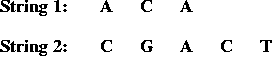• Match and gap scores, e.g.,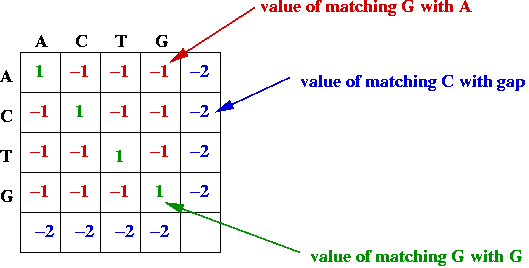• Goal: find the alignment with the best (largest) value.

• Potential asymmetry: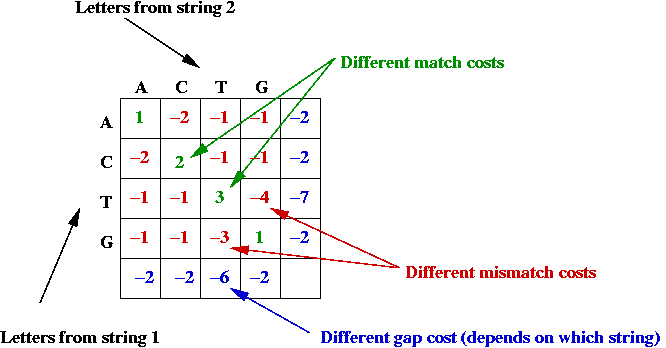• Some letter-alignments may be more favored.
• Some gap costs are different.

• Example: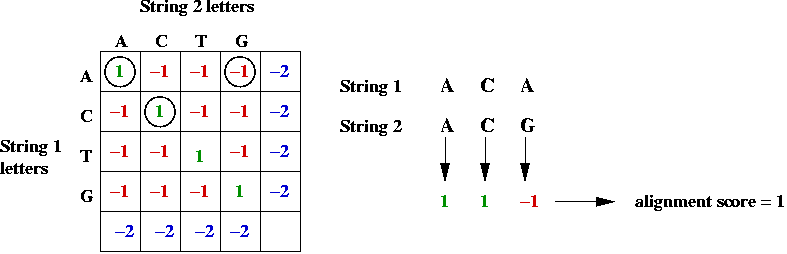• Example: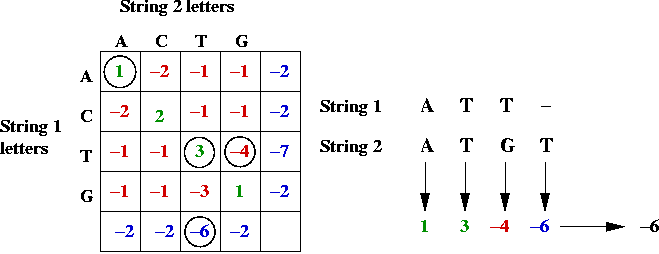Key ideas in solution:

• First, the alignment problem can be expressed as a graph problem: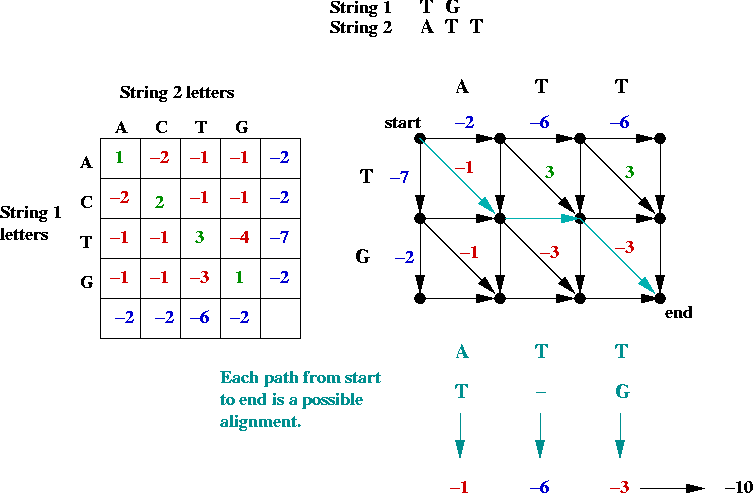• The diagonal edges correspond to character matches (whether identical or not).
• Each down edge corresponds to a gap in string 2.
• Each across edge corresponds to a gap in string 1.
• Goal: find the longest (max value) path from top-left to bottom-right.
=> longest-path problem in Directed Acyclic Graph.
• Can be solved in O(mn) time (using topological sort)
(m = length of string1, n = length of string2).
• Suppose we label the vertices (i, j) where i is the row, and j is the column.
• The best path to an intermediate node is via one of its neighbors: NORTH, WEST or NORTHWEST.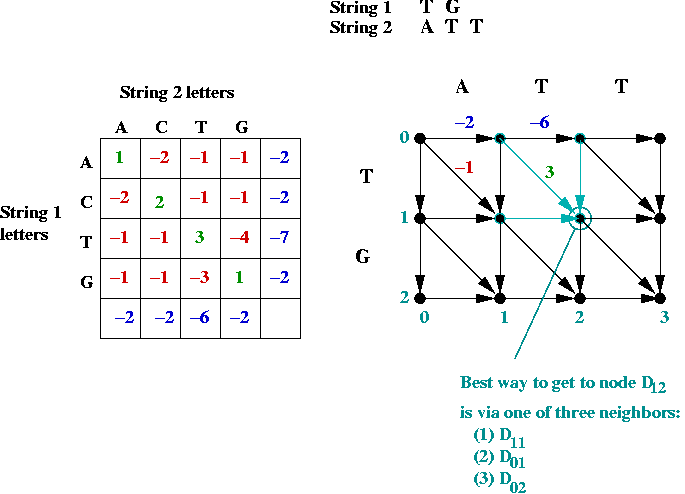• Dynamic programming approach:
• Building an actual graph (adjacency matrix etc) is unnecessary.
• Let Di,j
= value of best path to node (i,j) from (0, 0).
= score of optimal match of first i chars in string 1 to first j chars in string 2.
• Define (for clarity)  Ci-1,j-1 = Di-1,j-1 + exact-match-score Ci-1,j = Di-1,j + string1-gap-score Ci,j-1 = Di,j-1 + string2-gap-score
• Thus,  Di,j = max (Ci-1,j-1, Ci-1,j, Ci,j-1) = max (Di-1,j-1 + exact-match-score, Di-1,j + string1-gap-score, Di,j-1 + string2-gap-score)
• Initial conditions:
• D0,0 = 0
• Di,0 = Di-1,0 + gap-score for i-th char in string 1.
• D0,j = D0,j-1 + gap-score for j-th char in string 2.

Implementation:

• Pseudocode:
```Algorithm: align (s1, s2)
Input: string s1 of length m, string s2 of length n

1.    Initialize matrix D properly;

// Build the matrix D row by row.
2.    for i=1 to m
3.      for j=1 to n

// Initialize max to the first of the three terms (NORTH).
4.        max = D[i-1][j] + gapScore2 (s2[j-1])

// See if the second term is larger (WEST).
5.        if max < D[i][j-1] + gapScore1 (s1[i-1])
6.          max = D[i][j-1] + gapScore1 (s2[i-1])
7.        endif

// See if the third term is the largest (NORTHWEST).
8.        if max < D[i-1][j-1] + matchScore (s1[i-1], s2[j-1])
9.          max = D[i-1][j-1] + matchScore (s1[i-1], s2[j-1])
10.       endif

11.        D[i][j] = max

12.     endfor
13.   endfor

// Return the optimal value in bottom-right corner.
14.   return D[m][n]

Output: value of optimal alignment
```
• Explanation:
• The 2D array D[i][j] stores the Di,j values.
• The method matchScore returns the value in matching two characters.
• The method gapScore1 returns the value of matching a particular character in string 1 with a gap.
(gapScore2 is similarly defined).
• Note: D[i] and D[j] represent initial conditions.
• Note: there is one more row than the length of string 1.
(likewise for string 2).

• Computing the actual alignment:
• A separate 2D array tracks which term contributed to the maximum for each (i, j).
• After computing all of D, traceback and obtain path (alignment).

In-Class Exercise: (Solution) Download this template and implement the above algorithm. Gap and match costs are given.

Analysis:

• The double for-loop tells the story: O(mn) to compute Dm,n.

• Note: computing the actual alignment requires tracing back: O(m + n) time (length of a path).

• Space required: O(mn).

An improvement (in space):

• Genes are often longer than 10,000 bases (letters) long
=> space required is prohibitive.

• First, note that we only need successive rows in computing D
=> space requirement can be reduced to O(n).

• However, O(mn) space is still required to construct the actual alignment.

• A different approach:
• Consider the "middle" character in string 1
=> the character at position m / 2.
• In the optimal alignment, this character will either align with some j-th character in string 1, or a gap.
=> we can try all possible j values.
• To try these alignments, compute the m/2-th row (in O(mn) time).
• Output the optimal alignment found.
• Recurse on either side of the optimal alignment.

• It is possible to show: O(mn) time and O(n) space.

#### Local Alignment - The Smith-Waterman Algorithm

Consider a global alignment example: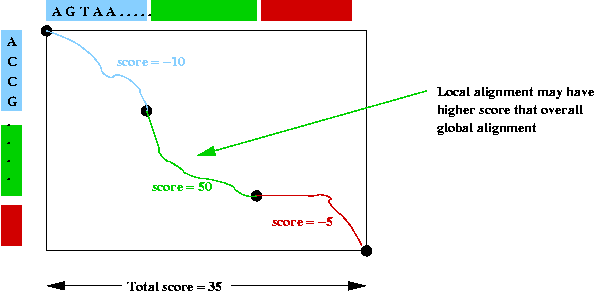• An alignment is forced across the whole table.
=> each position is scored.

• However, the score in one particular section may be higher
=> local alignments may have higher scores.

• Goal in local alignment: find best possible local alignment.

Two key modifications to global alignment algorithm:

1. Starting new alignments:
• As with global, start at top left corner.
• However, if an alignment gets too expensive, discard it and start a new one.

2. Look for best local alignment:
• As with global, fill all matrix entries.
• However, do not count on bottom-right as starting point
=> search entire matrix for best "bottom-right corner of local alignment"

To achieve the first part:

• Decide when a local alignment should be abandoned (i.e., when a new one should start).
=> Typically, when score goes below zero.

• Modify recurrence to avoid below-zero scores:  Di,j = max (0, Ci-1,j-1, Ci-1,j, Ci,j-1)

• If 0 is the maximum score at (i, j)
=> a new alignment is started.

• Record (i, j) values where new alignments start.

To achieve the second part:

• Search for Di,j with highest score.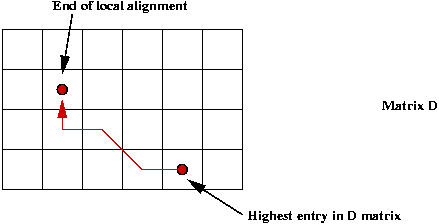• Work backwards from this point.

#### Scoring - the PAM Approach

What is PAM?

• PAM = Point Accepted Mutations.

• A theory for assigning scores to substitutions (mutations) in proteins.

Consider a group of related proteins and a particular position: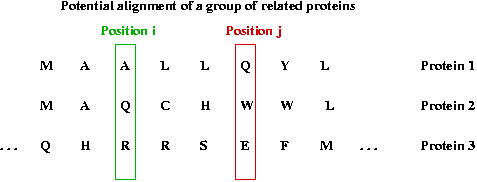• Suppose the proteins are listed in evolutionary order and aligned.

• We'll use a, b, c], ... to denote amino acids.

• A mutation from a to b can occur in one step or many.

• Let Mab = Pr[an a -> b mutation occurs] in some position.

• Let Maa = Pr[no mutation occurs].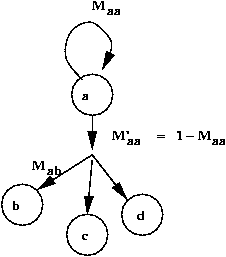• Let pa = Pr[residue a occurs].

Scoring intuition:

• Consider scoring the substitution a to b.

• Want a high score if Mab is high
=> because of high a -> b mutations.

• Want a low score if pb is high
=> because high occurence of b explains occurence of b (as opposed to mutation).

• Solution: let score be proportional to Mab / pb

PAM scoring:

• Consider mutations in two positions: a -> b and c -> d.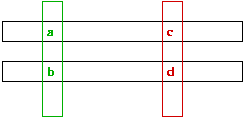• Probability of both occuring is proportional to product: (Mab / pb) * (Mcd / pd)

• But algorithm requires scores to be additive (for decomposability).

• Take logarithms:
score = log (Mab / pb) + log (Mcd / pd)

Evolutionary distance in PAM scoring:

• In comparing a -> b in two proteins:
• One possibility: the two proteins are phylogenetically adjacent
=> direct a -> b mutation.
• Another possibility: several intermediate mutations occured:
e.g., a -> e -> f -> b. (evolutionary distance = 3)
e.g., c -> g -> d. (evolutionary distance = 2)

• Goal: design scoring for a particular distance k.

• What do we want reflected in the score?
• Let Mabk = Pr[a -> b in k steps].
• Compute Mabk for each a, b.
• Score for a -> b is: log (Mabk / pb)

• Thus, in PAM-250 (a popular one), k = 250.

Note:

• We have only sketched some of the theory: a more rigorous explanation requires background in Markov chain theory.

• Usually, it is desirable to adjust the "rate of overall mutation" in the theory.
• Let M'aa = Pr[mutation from a]
• Let Maa = 1 - M'aa
• Adjust the ratio M'aa / Maa
• Typical value: 1/100
=> one mutation in every 100 amino acids.

#### How BLAST Works

Key ideas in BLAST (e.g, protein) searches:

• BLAST computes "similarity", not "alignment".

• Given two protein sequences:
• Find all substrings of length k (typical: k = 4) that occur in both strings.
• Build score based on matches.
• Extend substrings to see if match score can be increased.
• Compute total score when no more extensions are possible.

• Compute BLAST score against all proteins in database.

• Rank order search results by score.